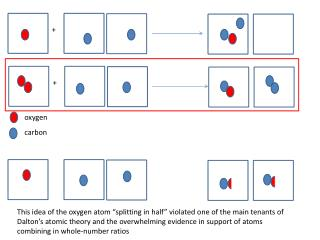DownloadDownload Presentationoxygen

# oxygen

Télécharger la présentation## oxygen

- - - - - - - - - - - - - - - - - - - - - - - - - - - E N D - - - - - - - - - - - - - - - - - - - - - - - - - - -
##### Presentation Transcript

1. + + oxygen carbon This idea of the oxygen atom “splitting in half” violated one of the main tenants of Dalton’s atomic theory and the overwhelming evidence in support of atoms combining in whole-number ratios

2. How Dalton Viewed Gases How Avogadro View These Gases Though it’s true 2 different gases have different atomic diameters, the space that gases occupy is so large compared to their atomic size that the size of each atom has no real affect on how many can fit into same volume

3. + + oxygen nitrogen

4. oxygen hydrogen Avogadro’s 2 key contributions: 1.) equal volumes of gases contain equal number of particles 2.) Some elemental gases are actually composed of more than 1 of the same type of atom (diatomic)

5. The importance of Avogadro’s hypothesis in determining relative mass 2 lbs 1 lb • Trying to determine relative mass this way isn’t useful or accurate • This suggests that the weight of a baseball is 1.2x greater than the weight of a basketball 5 lbs 4 lbs

6. The Importance of Avogadro’s Hypothesis in Determining Relative Mass 2 lbs 1 lb • This demonstrates that in order for us to properly determine relative mass, we need to make sure we’re talking about the same number of things if it’s truly going to be a fair and accurate comparison • This suggests that the weight of a single basketball is 2x greater than the weight of a baseball (which is true) 2 lbs 4 lbs

7. Once Avogadro’s hypothesis is accepted, we can do the exact same thing we did with basketballs and baseballs……..except with atoms!! • From there, we can determine the relative mass of certain elements • In order to do this, we need a reference element. Something that ALL the other elements are being compared to • The mass of all the other elements are RELATIVE to that one element we chose as a reference hydrogen • However much of an element occupied this much volume was referred to as a mole (mol) • Latin for “lump of stuff” 22.4 L

8. 1.99 g of hydrogen 27.91g of nitrogen 32.03 g of oxygen

9. Though this method of determining the relative mass of elements in the gas phase produced accurate results….. • It wasn’t very reproducible considering it was insanely hard, if not impossible, to accurately determine the density of many of the elements in the gas phase • So chemists started to use a different tool than comparing densities • Percent composition of compounds Because oxygen is so reactive, it was easy for chemists to compare the mass of several different elements with the same amount of oxygen when they formed compounds known as oxides (XO) For example: • 100 g of CO contains 42.9 g of C and 57.1 g of O • You learned how to arrive at these numbers last unit by calculating % composition and then figuring out the mass of each element in that compound If we’re going to compare the mass of several different elements based on how much of each reacts with oxygen, we better use the same amount of oxygen each time to be fair We could have chose any amount of oxygen but 100 is a nice and easy number so let’s just roll with that….

10. Mass of different elements that combine with 100 g of oxygen 6 7 8 27.92 100 107.92

11. But there appears to be a slight problem with our values compared to those in the periodic table • Dalton believed that the simplest way these elements could combine with oxygen is in a 1:1 ratio • Therefore, he believed that compounds, such as water, could be represented at HO • If we accept this formula for water, we end up concluding that oxygen is only 8x more massive than hydrogen • But here’s the problem…. Since volume ratios lead us to believe that water has 2 atoms of hydrogen for every 1 atom of oxygen, the problem with Dalton’s logic becomes obvious 12.5 200 • We came across this problem earlier • Need to have equal number of particles if you’re going to make an accurate comparison

12. Adjusting our previous table 6 12 7 14 8 16 27.92 55.8 100 200 107.9 215.8 The value for silver is 2x the accepted value on periodic table. This is due to the fact that it’s Ag2O

13. These new adjusted relative masses for each of the elements is now in agreement with what we see on the periodic table • Remember, these masses were all based on the idea of a mole • Therefore, we refer to these masses on the periodic table as their molar mass Molar mass: the mass of 1 mole of that particular element (g/mol) What is the molar mass of….. Sodium (Na): Cobalt (Co): Chlorine (Cl): Neon (Ne): • We can even go as far as determine the number of actual particles in a mole! • 6.02 x 1023 How many molecules are in….. 1 mole of oxygen: 16 g of oxygen :